# Calculus: Early Transcendentals: Chapter 5 Early Transcendentals Flashcards

Set Details Share
created 3 years ago by jerryberry
719 views
updated 3 years ago by jerryberry
Subjects:
mathematics
Page to share:
Embed this setcancel
COPY
code changes based on your size selection
Size:
X

1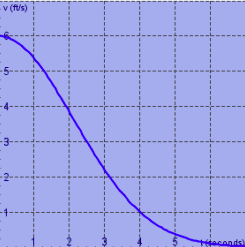The velocity graph of a braking car is shown. Use it to estimate to the nearest foot the distance traveled by the car while the brakes are applied. Use a left sum with n = 7.

19

2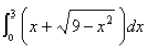Evaluate by interpreting it in terms of areas.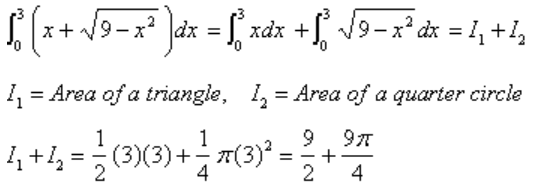3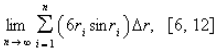Express the limit as a definite integral on the given interval.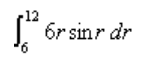4

Find an expression for the area under the graph of f as a limit. Do not evaluate the limit.

f(x) = √sinx x, 0 ≤ x ≤ π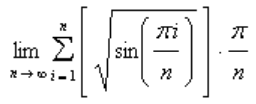5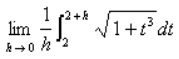Find the limit.

3

6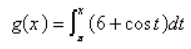Find gt(x) by evaluating the integral using Part 2 of the Fundamental Theorem and then differentiating.

6 + cos x

7

An animal population is increasing at a rate of 32 + 36t per year (where t is measured in years). By how much does the animal population increase between the fourth and tenth years?

1704

8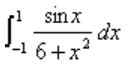Evaluate the definite integral.

0

9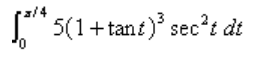Evaluate the integral.

18.75

10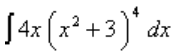Evaluate the indefinite integral.

2/5 (x2 + 3)5 + C

11

Use the definition of area to find the area of the region under the graph of f on [a, b] using the indicated choice of ck.

f(x) = x2, [0,6], ck is the left endpoint

72

12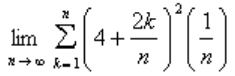Evaluate the limit after first finding the sum (as a function of n) using the summation formulas.

76/3

13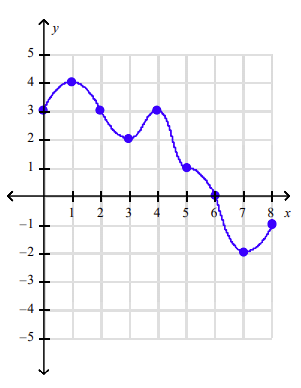The graph of a function f on the interval [0, 8] is shown in the figure. Compute the Riemann sum for f on [0, 8] using four subintervals of equal length and choosing the evaluation points to be (a) the left endpoints, (b) the right endpoints, and (c) the midpoints of the subintervals.

a. 18
b. 10
c. 10

14

You are given function f defined on an interval [a, b], the number n of subintervals of equal length ∆x = (b-a)/n, and the evaluation points ck in [xk -1, xk].
(a) Sketch the graph of f and the rectangles associated with the Riemann sum for f on [a, b], and
(b) find the Riemann sum.

f(x) = 2x - 3, [0,2], n=4, ck is the right endpoint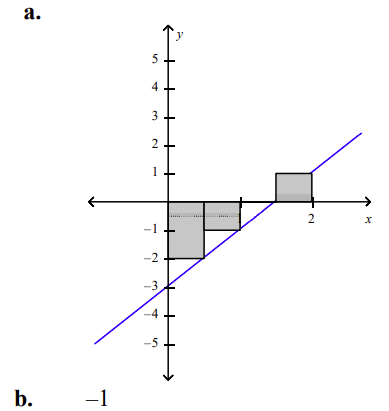15Evaluate

4

16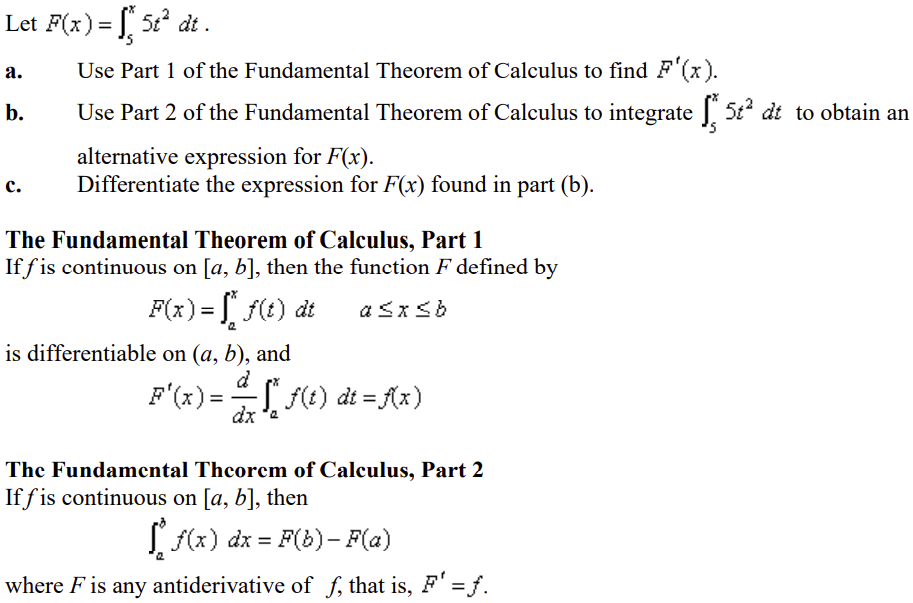a. 5x2
b. 5/3x3 - 625/3
c. 5x2

17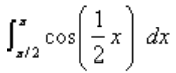Evaluate the integral.

2 - √2

18

The acceleration function of a body moving along a coordinate line is

a(t) = -7 cos 2t - 8 sin 2t t ≥ 0

Find its velocity and position functions at any time t if it is located at the origin and has an initial velocity of 4 m/sec.

v(t) = -7/2 sin 2t + 4 cos 2t, s(t) = 7/4 cos 2t + 2 sin 2t - 7/4

19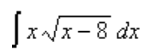Find the indefinite integral.

2/15(3x + 16)√(x-8)3 + C

20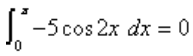Show by interpreting the definite integral geometrically.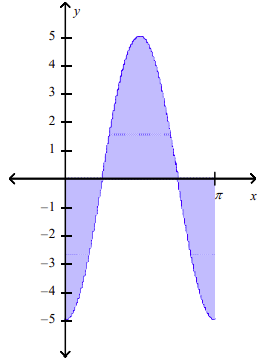A sketch of the area between the curve and the x-axis, shows that the area below the x-axis is equal to the area above the x-axis.

21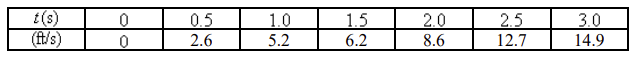The speed of a runner increased steadily during the first three seconds of a race. Her speed at half-second intervals is given in the table. Find a lower estimate for the distance that she traveled during these three seconds.

17.65

22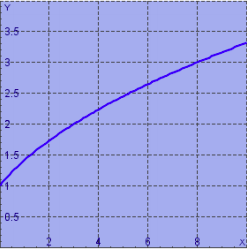By reading values from the given graph of f, use five rectangles to find a lower estimate, to the nearest whole number, for the area from 0 to 10 under the given graph of f.

21

23

Approximate the area under the curve y = 2/x2 from 1 to 2 using ten approximating rectangles of equal widths and right endpoints. Round the answer to the nearest hundredth.

0.93

24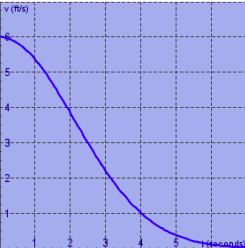The velocity graph of a braking car is shown. Use it to estimate to the nearest foot the distance traveled by the car while the brakes are applied. Use a left sum with n = 7.

19

25

Find an expression for the area under the graph of f as a limit. Do not evaluate the limit.

f(x) = √sin x, 0 ≤ x ≤ π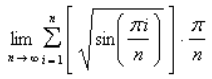26

If f(x) = √x-4, 1≤x≤6, find the Riemann sum with n = 5 correct to 3 decimal places, taking the sample points to be midpoints.

-10.857

27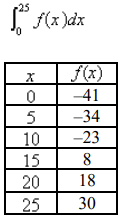A table of values of an increasing function f(x) is shown. Use the table to find an upper estimate of:

-210

28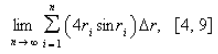Express the limit as a definite integral on the given interval.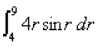29

Find the area of the region that lies under the given curve.

y = sin x, 0 ≤ x ≤ π/2

1

30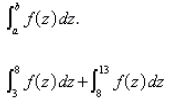Express the sum as a single integral in the form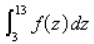31

Find an expression for the area under the graph of f as a limit. Do not evaluate the limit.

f(x) = √sin x, 0 ≤ x ≤ π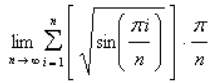32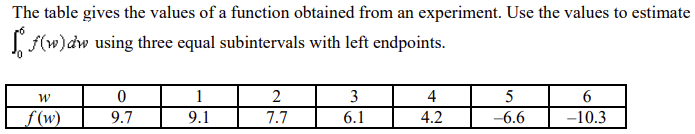43.2

33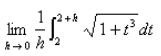Find the limit.

3

34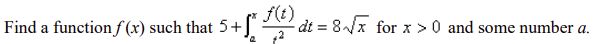f(x) = 4x3/2; for a = 25/64

35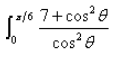Evaluate the integral.

7√3/3 + π/6

36

Find the area of the region that lies to the right of the y-axis and to the left of the parabola x = 3y - y2

9/2

37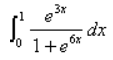Evaluate the integral.

1/3 arctan (e3) - π/12

38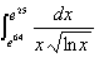Evaluate the definite integral.

-6

39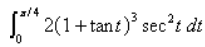Evaluate the integral.

7.5

40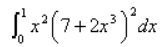Evaluate the integral.

193/9

41

Approximate the area under the curve y = sin x from 0 to π/2 using 8 approximating rectangles of equal widths and right endpoints. The choices are rounded to the nearest hundredth.
a. 3.09
b. 1.09
c. 4.09
d. 0.09
e. 2.09

b

42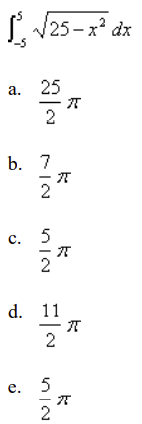Evaluate the integral by interpreting it in terms of areas.

a

43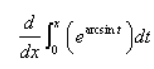Evaluate

a. earcsintx/4
b. earcsint
c. earcsinx
d. ex - arcsinx
e. e

c

44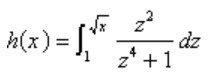Use Part 1 of the Fundamental Theorem of Calculus to find the derivative of the function.

a. √x+1/x2+2
b. √x2+1/2
c. √x/2(x2+1)
d. √x/x2+1
e. none of these

c

45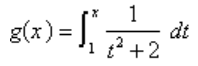Find the derivative of the function.

a. - 2x/(x2+2)2
b. -2/9
c. 1/3
d.1/x2+2

d

46

An animal population is increasing at a rate of 13 + 51t per year (where t is measured in years). By how much does the animal population increase between the fourth and tenth years?
a. 2220
b. 2240
c. 2270
d. 2320
e. 2230

a

47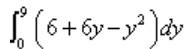Evaluate the integral.
a. 84
b. 94
c. 54
d. 34
e.74

c

48

The velocity function (in meters per second) is given for a particle moving along a line. Find the distance traveled by the particle during the given time interval.

v(t) = 8t - 8, 0 ≤ t ≤ 5

a. 36 m
b. 72 m
c. 100 m
d. 64 m
e. 68 m

e

49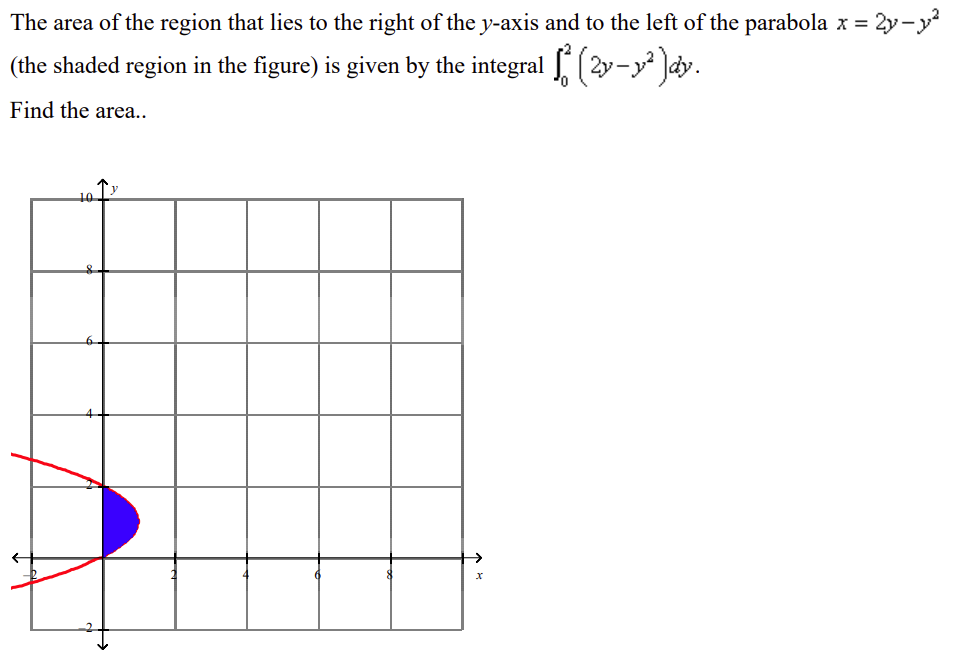a. 175/6
b. 4
c. 9
d. 4/3
e.6

d

50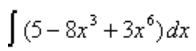Find the indefinite integral

a. 3/7 x7 - 2x4 + 5x + C
b. 5x - 8x4 + 3x7 + C
c. 3/7 x6 - 2x3 + 5x + C
d.5x - 24x2 + 18x5 + C

a

51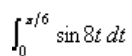Evaluate the definite integral.
a. 0.188
b. 3.25
c. -0.045
d. 0.35
e.2.891

a

52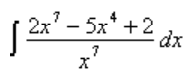Find the indefinite integral.

a. 2x + 5/4x4 - 1/4x8 + C
b. 2x + 5/2 x2 - 1/3 x6 + C
c. 2x + 5/4 x4 - 1/4 x8 + C
d. 2x + 5/2x2 - 1/3x6 + C

d

53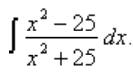Find the indefinite integral

a. x3-25x/x3+25x + C
b. x - 10 arctan(x/5) + C
c. x - 6 arctan(x/5) + C
d.x3-75x/x3+75x + C

b

54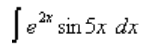Find the integral.
a. 1/29 e2x (2sin5x - 5cos5x) + C
b. 1/29 e2x (2sin5x - 5cos2x) + C
c. 1/29 e2x (2sin5x - 2cos5x) + C
d. 1/29 e2x (2sin5x - 5cos5x) + C

a

55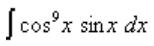Evaluate the indefinite integral.
a. 1/10 cot10x + C
b. 1/10 sin10x + C
c. 1/10 cos10x + C
d. - 1/10 sin10x + C
e. - 1/10 cos10x + C

e

56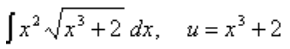Evaluate the integral by making the given substitution.
a. 2/3 (x3 + 2)3/2
b. 2/9 (x3 + 2)1/2 + C
c. - 2/9 (x3 + 2)3/2 + C
d. 2/9 (x3 + 2)3/2 + C
e. 1/9 (x3 + 2)1/2 + C

d

57

Evaluate the integral.

∫2 sin x cos(cos x) dx

a. -2cos(sin x) + C
b. sin(cosx)/2 + C
c. 2sin(cosx) + C
d. -2sin(cosx) + C
e. None of these

d

58

Find the integral.

∫tan3x sec5x dx

a. 1/7 sec7x - 1/5 sec5x + C
b. 1/5 sec5x + 1/3 sec3x + C
c. 1/7 sec7x + 1/5 sec5x + C
d. 1/5 sec5x - 1/3 sec3x + C

a

59

Find the integral using an appropriate trigonometric substitution.

∫x√9-x2 dx

a. 1/3 x2(9 - x2)3/2 + C
b. - 1/3 (9 - x2)3/2 + C
c. - 1/3 x2(9 - x2)3/2 + C
d. 1/3 (9 - x2)3/2 + C

b

60

Find the integral using an appropriate trigonometric substitution.

∫x3/√x2+36 dx

a. 1/3 (x2 + 36)3/2 √x2+36 + C
b. 1/3 (x2 + 72) √x2+36 + C
c. 1/3 (x2 - 72) √x2+36 + C
d. 1/3 (x2 - 36)3/2 √x2+36 + C

c

61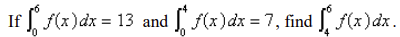a. 7
b. -13
c. 20
d. 6
e.13

d

62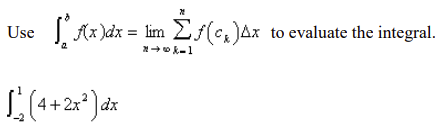a. 18
b. 22
c. 54
d. 22/3

a

63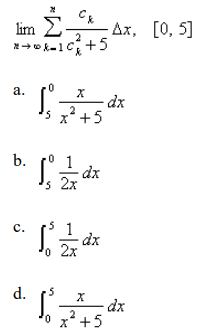The given expression is the limit of a Riemann sum of a function f on [a, b]. Write this expression as a definite integral on [a, b].

d

64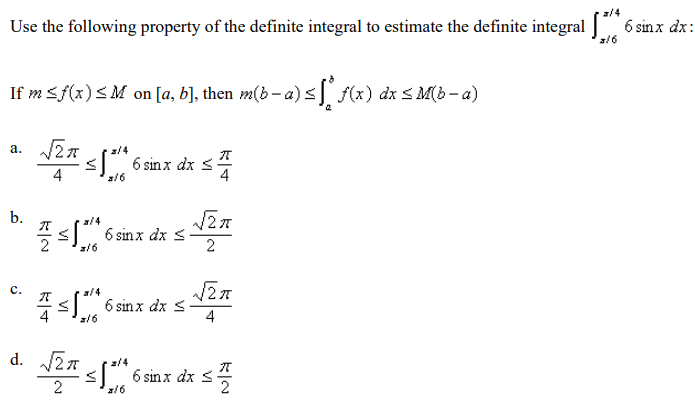c

65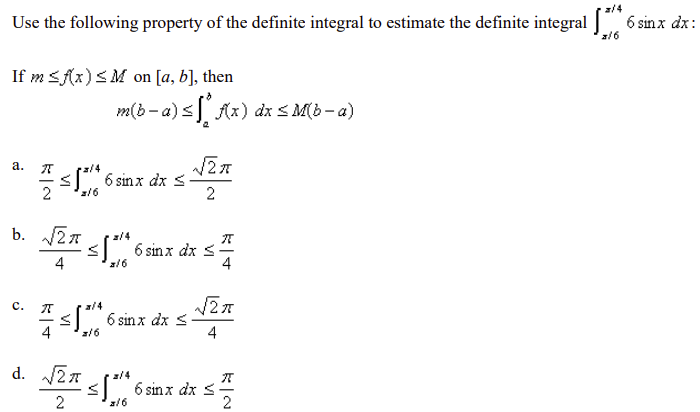c

66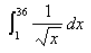Evaluate the integral.
a. –14
b. –11
c. 10
d. 14

c

67

The marginal cost of manufacturing x yards of a certain fabric is

Ct(x) = 3 - 0.01x + 0.000006x2

in dollars per yard. Find the increase in cost if the production level is raised from 1500 yards to 5500 yards.
a. \$188,000.00
b. \$198,000.00
c. \$178,000.00
d. \$218,000.00
e. \$208,000.00

b

68

The acceleration function (in m/s2) and the initial velocity are given for a particle moving along a line. Find the velocity at time t and the distance traveled during the given time interval.

a(t) = t + 4, v(0) = 6, 0 ≤t ≤ 10

a. v(t) = t2/2 + 6t m/s, 576 2/3 m
b. v(t) = t2/2 + 6t m/s, 601 2/3 m
c. v(t) = t2/2 +4t + 6 m/s, 526 2/3 m
d. v(t) = t2/2 +4t + 6 m/s, 426 2/3 m
e. v(t) = t2/2 + 6 m/s, 626 2/3 m

d

69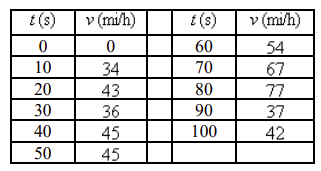The velocity of a car was read from its speedometer at ten-second intervals and recorded in the table. Use the Midpoint Rule to estimate the distance traveled by the car.

a. 1.2 miles
b. 1.8 miles
c. 0.8 miles
d. 2.4 miles
e. 0.6 miles

a

70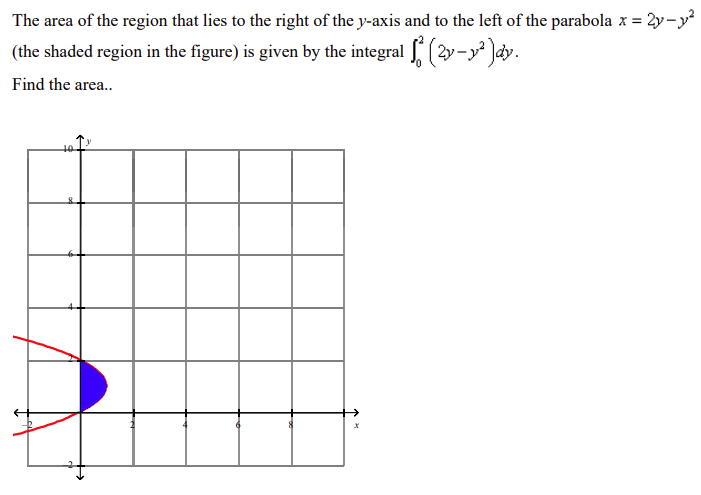a. 175/6
b. 4
c. 9
d. 4/3
e. 6

d

71

Find the area of the region under the graph of f on [a, b].

f(x) = x2 - 2x + 4; [-1,2]

a. 3
b. –12
c. 12
d. –3

c

72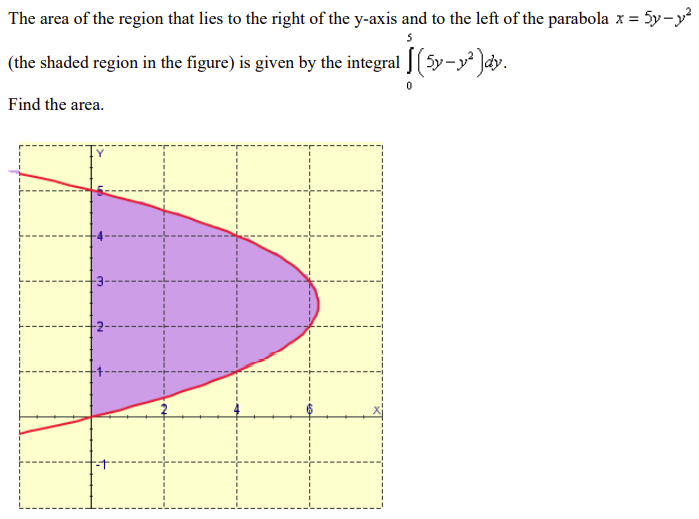a. 6.25
b. 125
c. 25/6
d. 125/6
e. 45.6

d

73

Find the indefinite integral.

∫(4 - 7x4 + 3x6)dx

a. 3/7 x7 - - 7/5 x5 + 4x + C
b. 4x - 28x3 + 18x5 + C
c. 3/7 x6 - 7/5 x4 + 4x + C
d.4x - 7x5 + 3x7 + C

a

74

Find the indefinite integral.

∫cot2x/cos2x dx

a. cos2x + C
b. tan x + C
c. csc2x + C
d.-cotx + C

d

75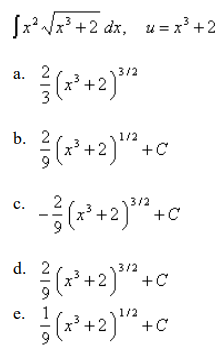Evaluate the integral by making the given substitution.

d

76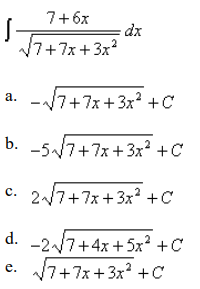Evaluate the indefinite integral.

c

77

Find the indefinite integral.

∫1-cos2x/sinx dx

a. sin x + C
b. 2x + C
c. -csc x + C
d.-cos x + C

d

78

Evaluate the integral.

∫2 sin x cos(cos x) dx

a. -2cos(sin x) + C
b. sin(cos x)/2 + C
c. 2sin(cos x) + C
d. -2sin(cos x) + C
e. None of these

d

79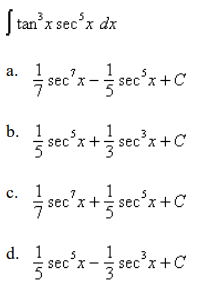Find the integral.

a

80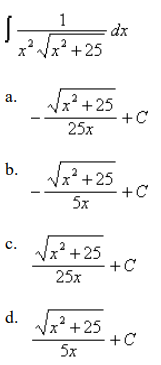Find the integral using an appropriate trigonometric substitution.

a

81

Estimate to the hundredth the area from 1 to 5 under the graph of f(x) = 5/x using four approximating rectangles and right endpoints. Select the correct answer.
a. 8.03
b. 10.03
c. 7.03
d. 9.03
e. 11.03
f. 6.03

a

82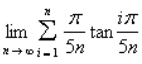Determine a region whose area is equal to

y = tan x, 0 ≤ x ≤ π/5

83

Approximate the area under the curve y = sin x from 0 to π/2 using 7 approximating rectangles of equal widths and right endpoints. The choices are rounded to the nearest hundredth.

1.11

84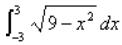Evaluate the integral by interpreting it in terms of areas.

9/2 π

85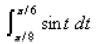Evaluate the integral. Select the correct answer.
a. 0.857
b. -0.943
c. 0.557
d. 0.057
e. 1.057

d

86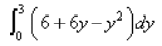Evaluate the integral.

36

87

Estimate the area from 0 to 5 under the graph of f(x) = 16 - x2 using five approximating rectangles and right endpoints. Select the correct answer.
a. 25
b. 20
c. 15
d. 125
e. 225

a

88

If ht is a child's rate of growth in pounds per year, which of the following expressions represents the increase in the child's weight (in pounds) between the years 3 and 6?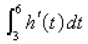89

Find the indefinite integral.

∫(8 - 7x3 + 4x6) dx

4/7 x7 - 7/4 x4 + 8x + C

90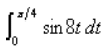Evaluate the definite integral. Select the correct answer.
a. -0.045
b. 0
c. 0.35
d. 3.25
e.2.891

b

91

Find the indefinite integral.

∫(9 - 7x3 + 4x7) dx

1/2 x8 - 74 x4 + 9x + C

92

Find the integral.

∫xe7x dx

1/49 (7x - 1)e7x + C

93

Find the integral using an appropriate trigonometric substitution.

∫x/√16-x2 dx

- √16-x2 + C

94

Find the integral.

∫x tan27x dx

1/7 x tan 7x + 1/49 ln|cos 7x| - 1/2 x2 + C

95

Evaluate the indefinite integral. Select the correct answer.

∫5ecos x sin x dx

a. -5sin(ecos x) + C
b. -ecos x sin x + C
c. -5ecos x + C
d. e5sin x + C
e. 5ecos x sin x + C

c

96

Find the area of the region that lies under the given curve. Round the answer to three decimal places.

y = √5x+2, 0 ≤ x ≤ 1

2.092

97

Find the indefinite integral. Select the correct answer.

2x(4x2 + 2)6 dx

a. 2x2(4x2 + 2)7 + C
b. 1/28 (4x2 + 2)7 + C
c. (4x2 + 2)7 + C
d. x2(4x2 + 2)7 + C

b

98

Find the integral.

∫sin5x cos4x dx

- 1/9 cos9x + 2/7 cos7x - 1/5 cos5x + C

99

Find the integral.

∫tan5x sec x dx

1/5 sec5x - 2/3 sec3x + sec x + C

100

Find the integral. Select the correct answer.

∫tan2x sec6x dx

a. 1/7 tan7x + 2/5 tan5x + 1/3 tan3x + C
b. 1/5 tan5x + 2/3 tan3x - tan x + C
c. 1/7 tan7x + 2/5 tan5x - 1/3 tan3x + C
d. 1/5 tan5x + 2/3 tan3x + tan x + C

a

101

Approximate the area under the curve y = sinx x from 0 to π/2 using 8 approximating rectangles of equal widths and right endpoints. The choices are rounded to the nearest hundredth.
a. 3.09
b. 1.09
c. 4.09
d. 0.09
e. 2.09

b

102

Evaluate the Riemann sum for f(r) = 6 - r2, 0 ≤ r ≤ 2 with four subintervals, taking the sample points to be right endpoints. Select the correct answer.
a. 10.75
b. 9.75
c. 8.25
d. 10.25
e. 9.25

c

103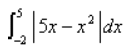Evaluate the integral.

33.5

104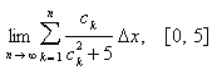The given expression is the limit of a Riemann sum of a function f on [a, b]. Write this expression as a definite integral on [a, b].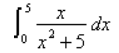105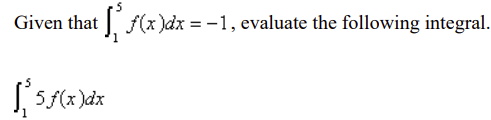-5

106

Find the area of the region that lies beneath the given curve. Select the correct answer.

y = sin x, 0 ≤ x ≤ π/3

a. 1.500
b. 1.450
c. –0.500
d. – 1.500
e. 0.500

e

107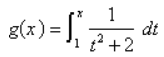Find the derivative of the function.

1/x2+2

108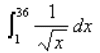Evaluate the integral. Select the correct answer.
a. –14
b. –11
c. 10
d. 14

c

109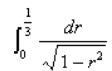Evaluate the integral.

0.340

110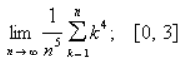Evaluate the limit by interpreting it as the limit of a Riemann sum of a function on the interval [a, b].

243/5

111

The marginal cost of manufacturing x yards of a certain fabric is

Ct(x) = 3 - 0.01x + 0.000006x2

in dollars per yard. Find the increase in cost if the production level is raised from 1500 yards to 5500 yards.
a. \$188,000.00
b. \$198,000.00
c. \$178,000.00
d. \$218,000.00
e. \$208,000.00

b

112

Evaluate the integral if it exists.

∫(5-x/x)2 dx

x - 10 ln x - 25/x + C

113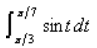Evaluate the integral.

-0.400

114

Find the indefinite integral.

∫(5 - 8x3 + 3x6) dx

3/7 x7 - 2x4 + 5x + C

115

Find the integral.

∫x sin 5x dx

1/25 (sin5x - 5x cos 5x) + C

116

Find the integral. Select the correct answer.

∫x tan25x dx

a. 1/2 x tan5x + 1/5 ln|cos5x| - 1/2 x2 + C
b. 1/2 x2 tan5x + 1/5 ln|cos5x| - 1/2 x2 + C
c. 1/5 x tan5x + 1/25 ln|cos5x| - 1/2 x2 + C
d. 1/5 x2 tan5x + 1/25 ln|cos5x| - 1/2 x2 + C

c

117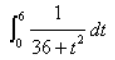Evaluate the integral.

π/24

118

Evaluate the indefinite integral.

∫7ecos x sin x dx

-7ecos x + C

119

Find the indefinite integral.

∫1-cos2x/sinx dx

-cos x + C

120

Find the integral.

∫tan3x sec5x dx

1/7 sec7x - 1/5 sec5x + C

121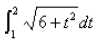Use the Midpoint Rule with n = 10 to approximate the integral. Select the correct answer.
a. 7.882848
b. 1.882848
c. 12.882848
d. 10.882848
e.2.882848

e

122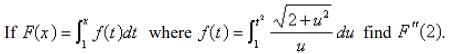3√2

123

Find the area of the region that lies beneath the given curve.

y = sin x, 0 ≤ x ≤ π/3

0.500

124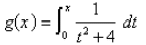Find the derivative of the function.

1/x2+4

125

Find the general indefinite integral. Select the correct answer.

∫sin40t/sin20t dt

a. -cos40t/40 + C
b. sin20t/10 + C
c. sin40t/40 + C
d. -sin40t/40 + C
e. cos40t/10 + C

b

126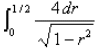Evaluate the integral.

2π/3

127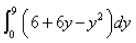Evaluate the integral. Select the correct answer.
a. 84
b. 54
c. 34
d. 74
e. 94

b

128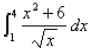Evaluate the integral.

24.4

129

If ht is a child's rate of growth in pounds per year, which of the following expressions represents the increase in the child's weight (in pounds) between the years 2 and 7?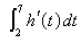130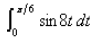Evaluate the definite integral.

0.188

131

Find the integral using an appropriate trigonometric substitution.

∫x/√4-x2 dx

-√4-x2 + C

132

Find the integral. Select the correct answer.

∫x7 ln x dx

a. 1/64 x8(ln x - 1) + C
b. 1/8 x7 + 1/x + C
c. 1/64 x8(8ln x - 1) + C
d. 1/8 x8(8ln x - 1) + C

c

133

Alabama Instruments Company has set up a production line to manufacture a new calculator. The rate of production of these calculators after t weeks is

dx/dt = 5700(1 - 140/(t+20)2)

calculators per week. Production approaches 5,700 per week as time goes on, but the initial production is lower because of the workers' unfamiliarity with the new techniques. Find the number of calculators produced from the beginning of the third week to the end of the fourth week. Round the answer to the nearest integer.

8377

134

Evaluate the indefinite integral.

∫cos8x sin x dx

- 1/9 cos9x + C

135

Evaluate the integral by making the given substitution.

∫x2 √x3+6 dx, u = x3 + 6

2/9 (x3 + 6)3/2 + C

136

Evaluate the indefinite integral. Select the correct answer.

∫7+12x/√7+7x+6x2 dx

a. 2 √7+7x+6x2 + C
b. - √7+7x+6x2 + C
c. -5 √7+7x+6x2 + C
d. √7+7x+6x2 + C
e. -2 √7+4x+5x2 + C

a

137

Find the integral using the indicated substitution.

∫tan6x sec2x dx, u = tan x

1/7 tan7x + C

138

Find the indefinite integral.

∫5x/√5-x2 dx

-5√5-x2 + C

139

Find the integral.

∫cos3x sin4x dx

1/5 sin5x - 1/7 sin7x + C

140

Find the integral.

∫tan4x sec4x dx

1/7 tan7x + 1/5 tan5x + C

141

Estimate to the hundredth the area from 1 to 5 under the graph of f(x) = 4/x using four approximating rectangles and right endpoints.

5.44

142

Find the area of the region under the graph of f on [a, b].

f(x) = x2 - 2x + 2; [-1,2]

6

143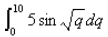Use the Midpoint Rule with n = 5 to approximate the integral. Select the correct answer. Round your answer to three decimal places.
a. 35.909
b. 37.709
c. 36.409
d. 31.409
e. 36.909

d

144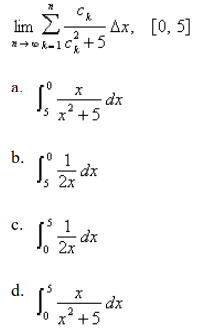The given expression is the limit of a Riemann sum of a function f on [a, b]. Write this expression as a definite integral on [a, b]. Select the correct answer.

d

145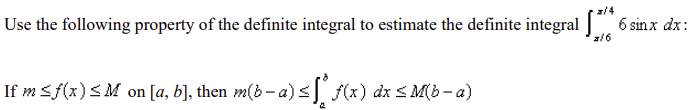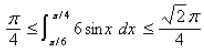146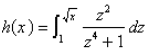Use Part 1 of the Fundamental Theorem of Calculus to find the derivative of the function.

√x/2(x2+1)

147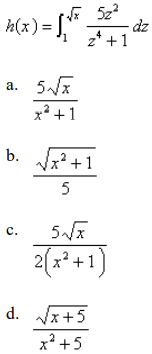Use Part 1 of the Fundamental Theorem of Calculus to find the derivative of the function. Select the correct answer.

c

148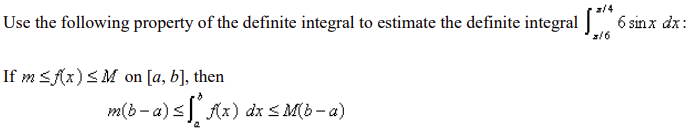149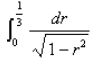Evaluate the integral. Select the correct answer.
a. 0.340
b. 0.111
c. 0.321
d. 0.987
e. 0.568

a

150

Find the general indefinite integral.

∫sin120t/sin60t dt

sin60t/30 + C

151

The marginal cost of manufacturing x yards of a certain fabric is

Ct(x) = 3 - 0.01x + 0.000006x2

in dollars per yard. Find the increase in cost if the production level is raised from 1500 yards to 5500 yards.

\$198,000.00

152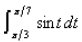Evaluate the integral. Select the correct answer.
a. –0.500
b. –1.000
c. -0.400
d. 0.250
e. 1.000

c

153

Find the indefinite integral.

∫(4 - 7x4 + 3x6) dx

3/7 x7 - 7/5 x5 + 4x + C

154

Find the integral.

∫x sin5x dx

1/25 (sin5x - 5xcos5x) + C

155

Alabama Instruments Company has set up a production line to manufacture a new calculator. The rate of production of these calculators after t weeks is

dx/dt = 5700(1 - 130/(t+18)2)

calculators per week. Production approaches 5,700 per week as time goes on, but the initial production is lower because of the workers' unfamiliarity with the new techniques. Find the number of calculators produced from the beginning of the third week to the end of the fourth week.

Round the answer to the nearest integer.
a. 8232
b. 7732
c. 8132
d. 8332
e. 8032

e

156

Evaluate the integral by making the given substitution.

∫x2 √x3+2 dx, u = x3 + 2

2/9 (x3 + 2)3/2 + C

157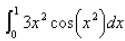Evaluate the integral if it exists.

none of these

158

Find the indefinite integral. Select the correct answer.

∫(x2 + 4x - 5)2(2x + 4) dx

a. -2(x2 + 4x - 5)3 + C
b. 2(x2 + 4x - 5)3 + C
c. (x2 + 4x - 5)3 + C
d. 1/3 (x2 + 4x - 5)3 + C

d

159

Evaluate the integral.

∫2sin x cos(cos x) dx

-2sin(cos x) + C

160

Find the integral using an appropriate trigonometric substitution. Select the correct answer.

∫x √9-x2 dx

a. 1/3 x2(9 - x2)3/2 + C
b. -1/3 (9 - x2)3/2 + C
c. -1/3 x2(9 - x2)3/2 + C
d. 1/3 (9 - x2)3/2 + C

b